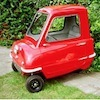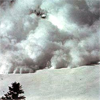#### You may also like### In the Playground

What can you say about the child who will be first on the playground tomorrow morning at breaktime in your school?### The Car That Passes

What statements can you make about the car that passes the school gates at 11am on Monday? How will you come up with statements and test your ideas?### Avalanche!

Investigate how avalanches occur and how they can be controlled

# Match the Matches

##### Age 7 to 11Challenge Level

Someone from Brenchley and Matfield School sent in this excellently reasoned solution - unfortunately he or she didn't give us their name:

Alpha united (AU) and Beta rovers (BR) played 15 games each.

• The pie chart and the bar chart had the same amount of goals.

• The tally chart and the pictogram had the same amount of goals.

• I then did this to find the mean of the pictogram: 0x3=0 1x3=3 2x2=4 3x5=15 4x2=8 0+3+4+15+8 = 30 Divided by 15 =2.

• I then found the mode which is 3 because 3 goals was scored mostly

• Finally I found the median which is 2. 000111223333344

• The mode is 1 higher than the mean and the median is the same as the mean.

• This matches the data of the writing about AU.

• This means the pictogram and the tally chart are AU.

• This means the pie chart and the bar chart are BR.

• AU scored 30 goals.

• BR scored 30 goals.

George and Dominic from St Nicholas' C of E School, Newbury, also sent in a very clear solution:

We looked at the pictogram and worked out how many goals were scored altogether - that was 30.
We divided it by 15 (the number of games) to find the average or mean - that was 2.
The tally chart goes with the pictogram as both have a mode of 3 scored goals. These two are both Alpha United because Alpha United's mode of the number of goals scored (3) is one more than the mean number of goals scored (2).

On the frequency chart, the most goals scored was one. When we added all of them together (30) and divided it by 15, we got 2. This is the mean.
For Beta Rovers, the mean number is one more than the mode number so the mode is one. This means the frequency chart must be Beta Rovers.

The pie chart shows that one goal was scored the most often and that 0 goals was scored the least. These facts also apply to the frequency chart. So the pie chart is also Beta Rovers.

So the tally chart and the pictogram were Alpha United, and the pie chart and frequency chart were Beta Rovers.

Here are some more solutions.

We started by looking at the pie chart, the mode was 1 goal, which was the same as the bar line graph. We knew that the pictograph and the tally chart matched because the mode is 3 on both. The mean is 2 for all 4 graphs. Alpha United has its mode one more than the mean, so Alpha United has to be grouped with the tally chart and the pictograph. Therefore Beta Rovers has to be with the pie chart and the bar line graph.
(Cirby, Isabella, George and Hannah, St Helen's C of E Primary School)

We started by ordering the numbers from the pictogram which were 0,0,0,1,1,1,2,2,3,3,3,3,3,4,4 and then calculated the median which was 2. We then calculated the mean which was 2. This was equal to the median which was one of the clues in the second box. We then used the chart to help calculate the mode by doing the reverse of the clue and adding 1 to 2 which was the mean and median.This gave us our mode which matched up to the clue we then repeated the process to calculate the other mean, median and mode this gave us our awnser. Alpha's data was the pictogram and the tally. Beta's was the pie chart and bar chart.
(Patrick and James, Redlands Primary School)

The first thing we did was pair the cards that had the same number of goals. We paired the tally chart with the pictogram and the pie chart with the bar line graph. Then we found out the mode of both pairs by looking at the tally and finding the most tally marks. We also looked at the pie chart and noticed the largest section. After that we found the mean. We had to time the number of goals by the number of times they came up, added the answers together and divided by 15 because they'd played 15 matches. We found the median by putting the numbers in order (eg for Alpha it was 000111223333344) and finding the middle number. Finally, we read the description and found which team fitted it. Alpha United was the team that had scored 3 goals 5 times and Beta Rovers was the team that had scored 1 goal 6 times.
(Grace and Katie, Greenacre School for Girls)

Stanburn Junior School sent us this.

Well done also to Amelia and Antonia from Greenacre School for Girls; Alice from Baston C of E Primary; Isaac and Happy from Glenview Primary, New Zealand; Charlotte; Laraib from Wellington; Wiktorial and Maiyam from St Paul's Catholic School; and Parallel Rangers from Wexham Court, who also sent good solutions.